Locating and Ordering Integers on the Number Line

Learning Outcomes

• Locate positive and negative numbers on a number line
• Compare and order positive and negative numbers using a number line

Do you live in a place that has very cold winters? Have you ever experienced a temperature below zero? If so, you are already familiar with negative numbers. A negative number is a number that is less than $0$. Very cold temperatures are measured in degrees below zero and can be described by negative numbers. For example, $-1^\circ F$ (read as “negative one degree Fahrenheit”) is $1$ degree below $0$. A minus sign is shown before a number to indicate that it is negative. The figure of a thermometer below shows $-20^\circ F$, which is $20$ degrees below $0$.

Temperatures below zero are described by negative numbers.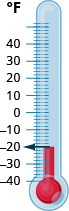Temperatures are not the only negative numbers. A bank overdraft is another example of a negative number. If a person writes a check for more than he has in his account, his balance will be negative.

Elevations can also be represented by negative numbers. The elevation at sea level is $0$ feet. Elevations above sea level are positive and elevations below sea level are negative. The elevation of the Dead Sea, which borders Israel and Jordan, is about $1,302$ feet below sea level, so the elevation of the Dead Sea can be represented as $-1,302$ feet. Refer to the image below for a depiction.

The surface of the Mediterranean Sea has an elevation of $0$ ft. The diagram shows that nearby mountains have higher (positive) elevations whereas the Dead Sea has a lower (negative) elevation.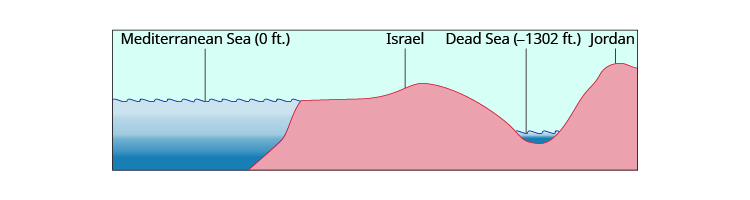Depths below the ocean surface are also described by negative numbers. A submarine, for example, might descend to a depth of $500$ feet. Its position would then be $-500$ feet as labeled in the image below.

Depths below sea level are described by negative numbers. A submarine $500$ ft below sea level is at $-500$ ft.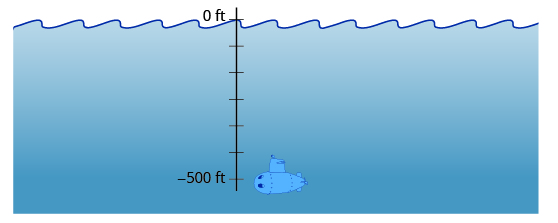Both positive and negative numbers can be represented on a number line. Recall that the number line created in Add Whole Numbers started at $0$ and showed the counting numbers increasing to the right as shown in the number line below. The counting numbers $(1, 2, 3, \ldots )$ on the number line are all positive. We could write a plus sign, $+$, before a positive number such as $+2$ or $+3$, but it is customary to omit the plus sign and write only the number. If there is no sign, the number is assumed to be positive.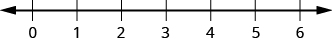Now we need to extend the number line to include negative numbers. We mark several units to the left of zero, keeping the intervals the same width as those on the positive side. We label the marks with negative numbers, starting with $-1$ at the first mark to the left of $0,-2$ at the next mark, and so on. Refer to the number line below for reference.

On a number line, positive numbers are to the right of zero. Negative numbers are to the left of zero. What about zero? Zero is neither positive nor negative.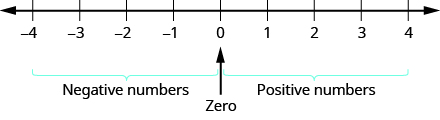The arrows at either end of the line indicate that the number line extends forever in each direction. There is no greatest positive number and there is no smallest negative number.

example

Plot the numbers on a number line:

1. $3$
2. $-3$
3. $-2$

Solution
Draw a number line. Mark $0$ in the center and label several units to the left and right.

1. To plot $3$, start at $0$ and count three units to the right. Place a point as shown in the number line below.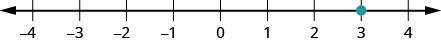2. To plot $-3$, start at $0$ and count three units to the left. Place a point as shown in the number line below.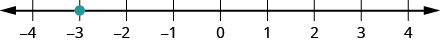3. To plot $-2$, start at $0$ and count two units to the left. Place a point as shown in the number line below.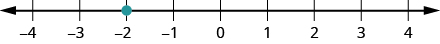try it

In the video below we show more examples of how to plot integers on a number line.

Order Positive and Negative Numbers

We can use the number line to compare and order positive and negative numbers. Going from left to right, numbers increase in value. Going from right to left, numbers decrease in value. See the number line below.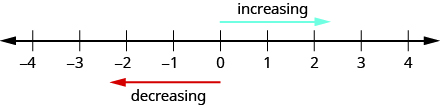Just as we did with positive numbers, we can use inequality symbols to show the ordering of positive and negative numbers. Remember that we use the notation $a<b$ (read $a$ is less than $b$ ) when $a$ is to the left of $b$ on the number line. We write $a>b$ (read $a$ is greater than $b$ ) when $a$ is to the right of $b$ on the number line. This is shown for the numbers $3$ and $5$ in the image below.

The number $3$ is to the left of $5$ on the number line. So $3$ is less than $5$, and $5$ is greater than $3$.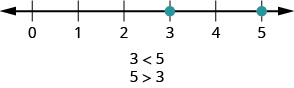The numbers lines to follow show a few more examples.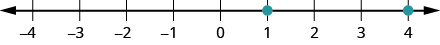$4$ is to the right of $1$ on the number line, so $4>1$.
$1$ is to the left of $4$ on the number line, so $1<4$.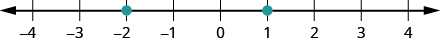$-2$ is to the left of $1$ on the number line, so $-2<1$.
$1$ is to the right of $-2$ on the number line, so $1>-2$.$-1$ is to the right of $-3$ on the number line, so $-1>-3$.
$-3$ is to the left of $-1$ on the number line, so $-3<-1$.

example

Order each of the following pairs of numbers using $<$; or $\text{>:}$

$14$ and $6$
$-1$ and $9$
$-1$ and $- 4$
$2$ and $- 20$

try it

In the video below we show more examples of how to use inequality symbols to compare integers.